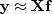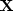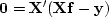Next: Unknown input: deconvolution with Up: Time domain versus frequency Previous: Time domain versus frequency

### Unknown filter

Given inputs and outputs, the problem of finding an unknown filter appears to be overdetermined, so we writewhere the matrixis a matrix of downshifted columns like (19). Thus the quadratic form to be minimized is a restatement of equation (25) using filter definitions:(27)
The solutionis found just as we found (26), and it is the set of simultaneous equations.Next: Unknown input: deconvolution with Up: Time domain versus frequency Previous: Time domain versus frequency
Stanford Exploration Project
10/21/1998